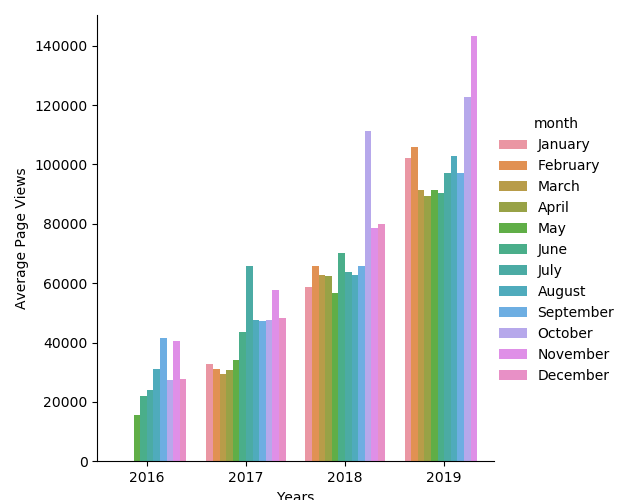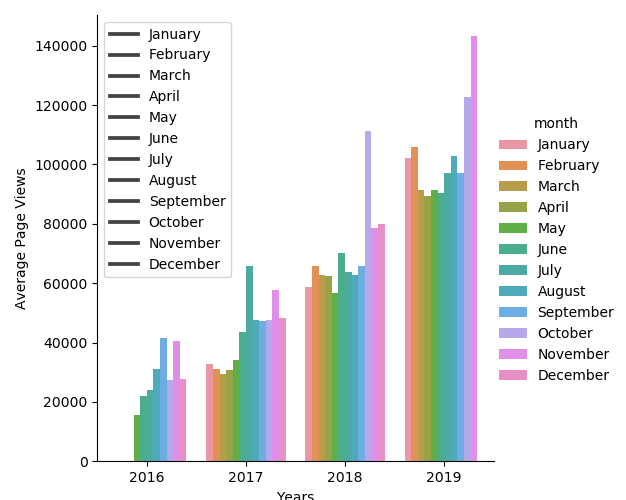# Page View Time Series - Code Optimisations

Hi there everyone

I have now completed the Page View Time Series Visualizer challenge and handed my code in.

There are a couple of sections of my code however that I think could possibly be improved upon, both within the `draw_bar_plot()` function. The code for which is here:

def draw_bar_plot():
# Copy and modify data for monthly bar plot
df_bar = df.copy()
df_bar = df_bar.reset_index(level=[‘date’])
df_bar = df_bar.assign(year = lambda x: (x[‘date’].dt.strftime(’%Y’)))
df_bar[‘month’] = [get_month(x) for x in df_bar[‘date’].dt.strftime(’%m’)]
df_bar = df_bar.drop(columns=[‘date’])

``````# Create dataframe for monthly average values
column_names = ['year', 'month', 'average']
df_aver = pd.DataFrame(columns = column_names)
year = None
month = None
first = True  # Flag to indicate if this will be the first row in our dateframe
total = 0  # Total number of page views in the current month
count = 0  # Total number of days parsed for the current month
for row in df_bar.itertuples():
if (row == year) & (row == month):
count += 1
total += row
else:
# New entry in df_aver dataframe
if first is True:  # So we initialise first entry
year = row
month = row
count += 1
first = False
else:
# We have a new month, append previous month to df_aver
average = round(total / count, 1)
df_aver = df_aver.append({'year' : year, 'month' : month, 'average' : average}, ignore_index=True)
# Reset variables for new month
year = row
month = row
count = 1
total = row

# Draw bar plot
fig = sns.catplot(x="year", y="average", hue="month", kind="bar", data=df_aver,
hue_order=['January', 'February', 'March', 'April', 'May', 'June', 'July', 'August',
'September', 'October', 'November', 'December']).fig
plt.legend(labels=('January', 'February', 'March', 'April', 'May', 'June', 'July', 'August',
'September', 'October', 'November', 'December'),
loc='upper left', bbox_to_anchor=(0, 1))
plt.xlabel("Years")
plt.ylabel("Average Page Views")

# Save image and return fig (don't change this part)
fig.savefig('bar_plot.png')
return fig
``````

The first section I think could possibly be optimised is the part beginning with the comment `Create dataframe for monthly average values`, to do this I iterate through each row in the `df_bar` dataframe using `itertuples()` and create a new dataframe containing a row for each month/year and an average for that month. My question being, is there a more efficient or succinct way to do this? I have read comments stating that it is best not to iterate through dataframes if at all possible, however I have not been able to think of a functioning alternative at this point.

The second section is where the actual plot is drawn. I used `sns.catplot()` to do this and originally I did this without using the `legend()` method.

fig = sns.catplot(x=“year”, y=“average”, hue=“month”, kind=“bar”, data=df_aver,
hue_order=[‘January’, ‘February’, ‘March’, ‘April’, ‘May’, ‘June’, ‘July’, ‘August’,
‘September’, ‘October’, ‘November’, ‘December’]).fig
plt.xlabel(“Years”)
plt.ylabel(“Average Page Views”)

which creates what I think is a nice plot that closely resembles the example (although the legend is in the wrong place).In this state however it failed one of the tests because it doesn’t have a specific legend. By adding a legend I was able to pass the test but the plot no longer looks nice.

plt.legend(labels=(‘January’, ‘February’, ‘March’, ‘April’, ‘May’, ‘June’, ‘July’, ‘August’,

``````                             'September', 'October', 'November', 'December'),

loc='upper left', bbox_to_anchor=(0, 1))
``````By passing the test in this manner I feel I have ‘hacked’ it somewhat, any advice on how I could tidy up the plot yet still pass the test would be greatly appreciated.

My apologies for the long post but I thought these would be interesting questions for the community.

I think I figured all this out. Turns out I had the same issues you had but I rode right past them because I passed the tests. I’m going to answer things in reverse order because that turns out to be the logical sequence.

Your last plot is the same plot I had that passed. The legend on the right is generated by `sns.catplot()` and the left by `plt.Axes.legend()`. The legend tests expect the latter; I suspect the example was generated by matplotlib entirely and not seaborn. I generated your first plot with `graph = sns.catplot()` and some label editing. By playing around with `dir()` and the `FacetGrid` returned by `sns.catplot()` interactively, I found the seaborn legend at `graph.legend` and could access its labels like the tests would, but the tests expect to be able to access the plot’s x and y axis labels too, which are all available on the `plt.Axes.legend()` version. So the choice was to either rewrite all the tests or hide the seaborn legend; so I hid it. Summarizing how to get stuff where it belongs:

``````# Create the plot.
graph = sns.catplot(...)
graph.despine(left=True)
graph.set_axis_labels('Years', 'Average Page Views')
graph.legend.set_title('')
# Remove the very nice seaborn legend.
graph._legend.remove()

# The fig is at graph.fig.  Not at all confusing.
fig = graph.fig
# Create the new legend.
fig.axes.legend()

#Save the plot.
fig.savefig(...)

# Return the fig for testing.
return fig
``````

Finally, your suspicions about the data massage are correct. The easiest way to shorten it is to look at the documentation on the pandas time functions, like `dt.year` and `dt.month` and also `.mean()`.

Thanks for reminding me I had this problem too. You got the data and plots in the right places to pass the tests, regardless of how you did it, even if the tests and seaborn can’t agree on where to put legends and axis labels.

Hi sorry I haven’t replied yet I’ve been busy doing other stuff over the past week (HTML and Django). Thanks for spending the time looking at my code it is much appreciated, I’ll have a go at these changes soon.